Exponentials

 Site: TBAISD Moodle Course: Michigan Algebra II Preview 2012 Book: Exponentials Printed by: Guest user Date: Sunday, July 5, 2020, 04:52 AM

Growth

The exponential parent function is y = kx where k is a constant. The easiest way to begin looking at this pattern is to make a table.

Recall from the Families of Functions Unit that the function g(x) = 2x would create the following table and graph: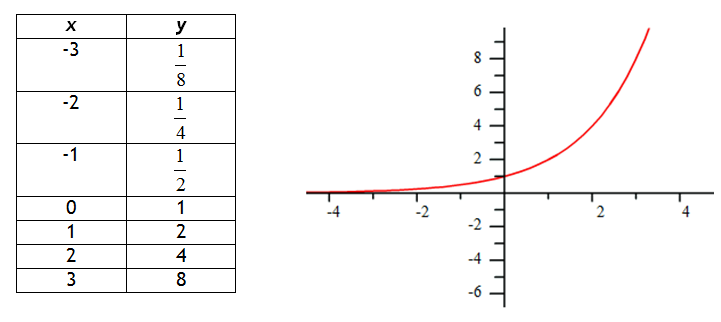Notice that the graph increases more rapidly as x increases. The left side of the graph is approaching, but never touches the line y = 0, which is the graph's asymptote. Therefore, the domain of this function is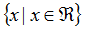and the range of the function is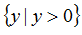.

Decay

Recall that the function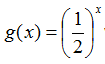would create the following table and graph: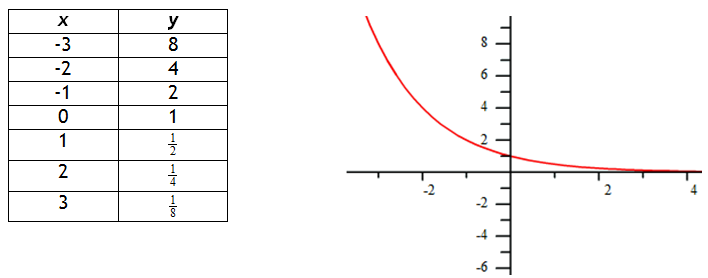Notice that the graph decreases more rapidly as x increases. The right side of the graph is approaching, but never touches the line y = 0, which is the graph's asymptote. Therefore, the domain of this function is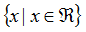and the range of the function is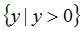.

Video Lesson: Graphing

Interactive Activity

Transformations

The transformations of functions that were presented in the Families of Functions unit also apply to exponential functions. The following list shows how the patterns apply to exponentials:

1. y = 2 x + a translates the graph a units up; asymptote equation y = a

2. y = 2 x -a translates the graph a units down; asymptote equation y = -a

3. y = 2 x-a translates the graph a units right; asymptote equation y = 0

4. y = 2 x+a translates the graph a units left; asymptote equation y = 0

5. y=-(2 x) reflects the graph across the x-axis; asymptote equation y = 0

6. y = 2 -x reflects the graph across the y-axis; asymptote equation y = 0

Video Lessons

To learn how to transform exponential graphs, select the following link:

Video Lesson:

To see more examples on exponential transformations, view the video below.

Interactive Activity

Exponential Functions

Identifying

Exponential Functions follow the form y = abx where a is the y-intercept and b is the common ratio of the table. When modeling exponential data the a-value is the y-intercept, therefore find the point where x = 0. To determine the b-value calculate the common ratio between consecutive y-values.

Video Lesson

To learn how to identify exponential data, select the following link:

Modeling A Graph

In order to write the function that models an exponential graph, find at least two points on the graph. If the y-intercept (0, a) is easily determined, select it as one of the two points. Otherwise, select two points with integer coordinates to make the computation easier. Then calculate the a and b-values.

Example Write a function to model the graph.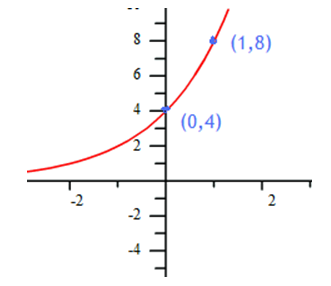Step 1. Determine the y-intercept.

Notice (0, 4) is on the graph, therefore a = 4 and y = 4 . bx

Step 2. Determine another point on the graph and substitute it into x and y in the equation, then solve for b.

Notice (1, 8) is on the graph, use it in the equation.

8 = 4 . b1

2 = b

Therefore, y = 4 . 2x models this graph.

Modeling Points

When given two or more points of an exponential graph, try one of the following methods:

• If the y-intercept is one of the points, the model can be found exactly like the previous example.
• If the y-intercept is not given, put the points into a table and find the common ratio.

Example Find the exponential function that models the points (2, 6) and (4, 54)

Step 1. Put the points into a table.

 x y 0 1 2 6 3 4 54

Step 2. Find the common multiplier between the y-values.

6 . __ = 54

6 . 9 = 54

Example Continued

Step 3. Find the common ratio.

The common ratio is based on x-values with an increment of 1. If the increment is not 1, then set up the equation
b x-increment = common multiplier and solve for b.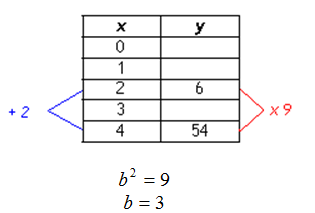Step 4. Use b to fill in the rest of the table.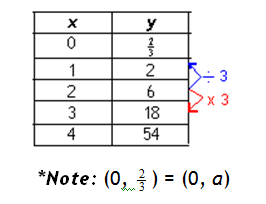Step 5. Write the exponential model.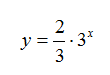Guided Practice

To solidify your understanding of identifying and modeling exponential data, visit the following link to Holt, Rinehart, and Winston Homework Help Online. It provides examples, video tutorials and interactive practice with answers available. The Practice and Problem Solving section has two parts. The first part offers practice with a complete video explanation for the type of problem with just a click of the video icon. The second part offers practice with the solution for each problem only a click of the light bulb away.

Guided Practice

Sources

Sources used in this book:

Edwards, P. "The Exponential Function Applet." http://mathinsite.bmth.ac.uk/applet/exponential/exp.html (accessed 7/13/2010).

Embracing Mathematics, Assessment & Technology in High Schools; a Michigan Mathematics & Science Partnership Grant Project

"Exponential Growth Equations and Graphs ." http://www.mathwarehouse.com/exponential-growth/graph-and- equation.php (accessed 7/13/2010).

Holt, Rinehart & Winston, "Exponential and Logarithmic Functions." http://my.hrw.com/math06_07/nsmedia/homework_help/alg2/alg2_ch 07_08_hom eworkhelp.html (accessed 7/13/2010).

Holt, Rinehart & Winston, "Identifying Exponential Data." http://my.hrw.com/math06_07/nsmedia/lesson_videos/alg2/player.ht ml?contentSr c=6468/6468.xml (accessed 7/13/2010).

Holt, Rinehart & Winston, "Transformations of Exponential Functions." http://my.hrw.com/math06_07/nsmedia/lesson_videos/alg2/player.ht ml?contentSr c=7157/7157.xml (accessed 7/13/2010).

Holt, Rinehart & Winston, "Translating Exponential Functions." http://my.hrw.com/math06_07/nsmedia/lesson_videos/alg2/player.ht ml?contentSr c=6467/6467.xml (accessed 7/13/2010).